Algebra I: Ratios, Proportions and Equivalence Classroom ActivitiesDownload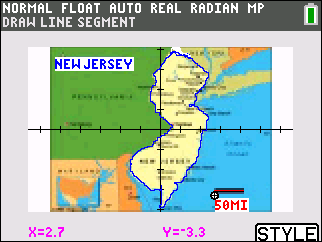Mapping Success

In this activity, students will load a background image of a map and use the scale on the map to estimate distances.
•TI-84 Plus CE
•TI-84 Plus C Silver Edition
• TI-84 Plus Silver Edition
• TI-84 Plus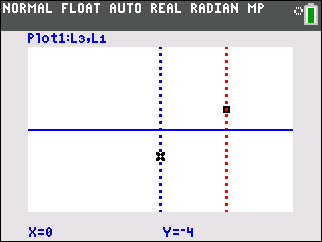Hot Air Balloon

Using a dynamic manipulative, students will have a visual for adding and subtracting integers.
•TI-84 Plus CE
•TI-84 Plus C Silver Edition
• TI-84 Plus Silver Edition
• TI-84 Plus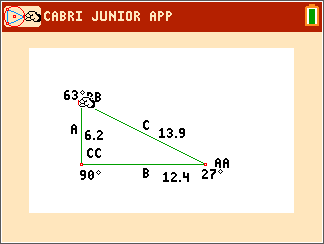Trigonometric Ratios

Students discover the trigonometric ratios through measuring the side lengths of similar triangles and calculating their ratios. The formal definitions of the sine, cosine, and tangent or an angle are presented and applied to find the missing side lengths.
•TI-84 Plus CE
•TI-84 Plus C Silver Edition
• TI-84 Plus Silver Edition
• TI-84 PlusCommon Denominator

Students will review and practice adding fractions with unlike denominators.
•TI-84 Plus CE
•TI-84 Plus C Silver Edition
• TI-84 Plus Silver Edition
• TI-84 Plus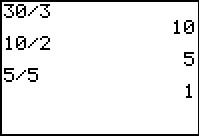Factoring Composite Numbers

Students will review some of the terms associated with prime factors. A Frayer Model (Square) is provided allowing the teacher to assess students’ knowledge of the concept prime.
•TI-84 Plus CE
•TI-84 Plus C Silver Edition
• TI-84 Plus Silver Edition
• TI-84 Plus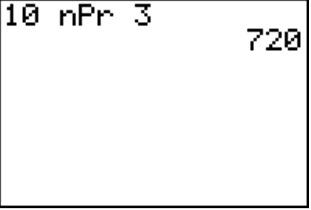Perms and Combs?

Students will use built-in commands to evaluate factorials, combinations, and permutations.
•TI-84 Plus CE
•TI-84 Plus C Silver Edition
• TI-84 Plus Silver Edition
• TI-84 Plus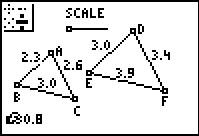Ratios of Similar Triangles

Students explore two ways of comparing side lengths of similar triangles. They will calculate ratios and change the triangles to see how the ratio changes.
• TI-84 Plus Silver Edition
• TI-84 PlusSupertall Skyscrapers

Students measure scale drawings of famous "supertall" skyscrapers and solve more proportions to find the heights of other skyscrapers drawn with the same scale.
• TI-84 Plus Silver Edition
• TI-84 Plus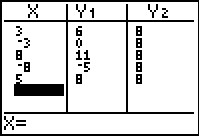One Step at a Time

Students solve one-step equations involving addition and multiplication by substituting possible values of a variable.
•TI-84 Plus CE
•TI-84 Plus C Silver Edition
• TI-84 Plus Silver Edition
• TI-84 Plus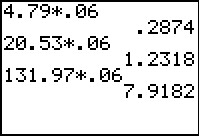••